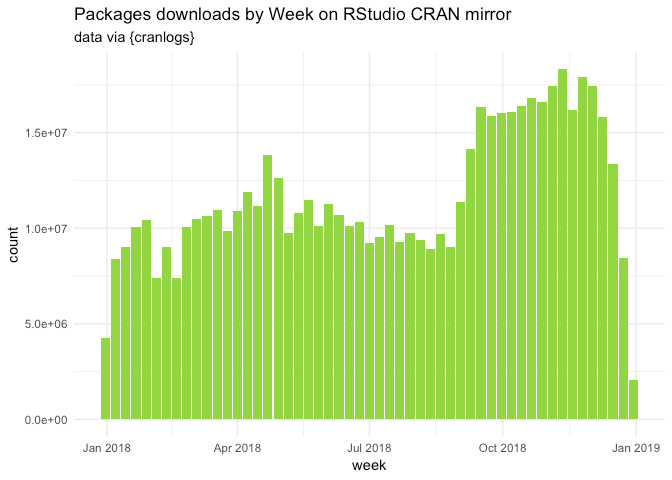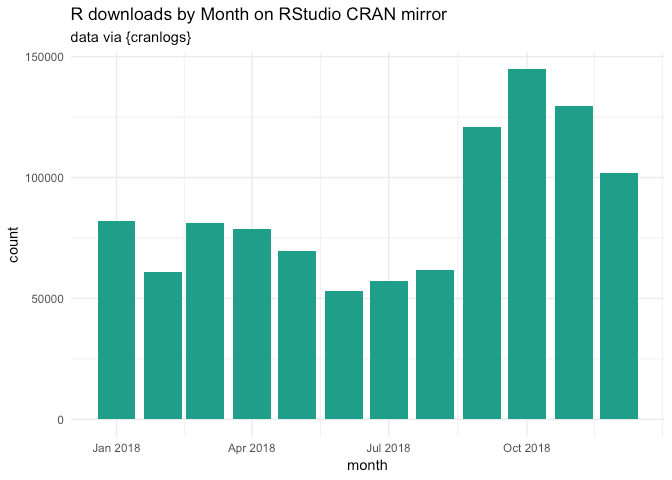Want to share your content on R-bloggers? click here if you have a blog, or here if you don't.

2018 at glance with `{cranlogs}`.

``````library(cranlogs)
library(data.table)
library(lubridate)
``````
``````##
## Attaching package: 'lubridate'

## The following objects are masked from 'package:data.table':
##
##     hour, isoweek, mday, minute, month, quarter, second, wday,
##     week, yday, year

## The following object is masked from 'package:base':
##
##     date
``````
``````library(ggplot2)
library(magrittr)
``````

We’ll use `{cranlogs}` to retrieve the data from the RStudio CRAN
mirror.

``````total_dl <- cran_downloads(from = "2018-01-01", to = "2018-12-31")

# Turn to a data.table
setDT(total_dl)

# Round the date to month and week
total_dl[, `:=`(
round_week = floor_date(date, "week" ),
round_month = floor_date(date, "month" )
) ]
``````

``````total_dl[, .(total = sum(count))]
``````
``````##        total
## 1: 614548197
``````

Let’s plot this:

``````random_viridis <- function(n){
sample(viridis::viridis(100), n)
}
total_dl[, .(count = sum(count)), round_week] %>%
ggplot(aes(round_week, count)) +
geom_col(fill = random_viridis(1)) +
labs(
subtitle = "data via {cranlogs}",
x = "week"
) +
theme_minimal()
````````````total_dl[, .(count = sum(count)), round_month] %>%
ggplot(aes(round_month, count)) +
geom_col(fill = random_viridis(1)) +
labs(
subtitle = "data via {cranlogs}",
x = "month"
) +
theme_minimal()
``````Let’s now have a look at the number of downloads for R itself:

``````total_r <- cran_downloads("R", from = "2018-01-01", to = "2018-12-31")

setDT(total_r)

total_r[, `:=`(
round_week = floor_date(date, "week" ),
round_month = floor_date(date, "month" )
) ]
``````

``````total_r[, .(total = sum(count))]
``````
``````##      total
## 1: 1041727
``````

Plotting this:

``````total_r[, .(count = sum(count)), round_week] %>%
ggplot(aes(round_week, count)) +
geom_col(fill = random_viridis(1)) +
labs(
subtitle = "data via {cranlogs}",
x = "week"
) +
theme_minimal()
````````````total_r[, .(count = sum(count)), round_month] %>%
ggplot(aes(round_month, count)) +
geom_col(fill = random_viridis(1)) +
labs(
subtitle = "data via {cranlogs}",
x = "month"
) +
theme_minimal()
``````version:

``````total_r[, .(count = sum(count)), version][order(count, decreasing = TRUE)] %>%
``````
``````##          version  count
##  1:        3.5.1 464837
##  2:        3.4.3 174665
##  3:        3.5.0 137886
##  4:        3.4.4 107124
##  5:       latest  32642
##  6: 3.5.1patched  32119
##  7:        3.3.3  27992
##  8:        3.5.2  21645
##  9:        devel   8543
## 10:        3.2.4   4814
``````
``````total_r[, .(count = sum(count)), version][order(count)] %>%
``````
``````##        version count
##  1:    3.5.1rc     2
##  2:  3.5.2beta     2
##  3:    3.5.2rc     6
##  4:  3.5.0beta     8
##  5:    3.4.4rc    11
##  6: 3.5.0alpha    11
##  7:    3.5.0rc    12
##  8:      2.6.1    13
##  9:      2.8.0    16
## 10:      2.2.1    17
``````
``````total_r[, .(count = sum(count)), version][order(count, decreasing = TRUE)] %>%
ggplot(aes(reorder(version, count), count)) +
coord_flip() +
geom_col(fill = random_viridis(1)) +
labs(
title = "10 most downloaded R versions in 2018 on RStudio CRAN mirror",
subtitle = "data via {cranlogs}",
x = "version"
) +
theme_minimal()
``````And by os:

``````total_r[, .(total = sum(count)), os]
``````
``````##     os  total
## 1: osx 228573
## 2: win 767319
## 3: src  42725
## 4:  NA   3110
``````

And a happy new year 🎉🎉